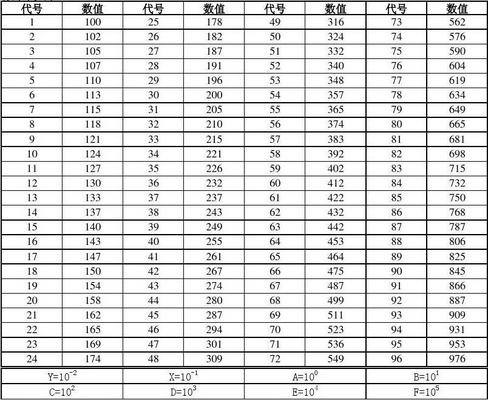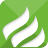40年专注研发生产集一体的电阻元器件供应链

## 联系我们## 精密电阻阻值对照表

### 文章来源：插件电阻人气：10386发表时间：2018-11-07 14:39:34【小中大】

众所周知，精密电阻一般是指精度高(万分之一以上)、温漂低(10ppm以下)及长期稳定性(年变化率小于50ppm)的电阻，它相比于金属膜电阻、线绕电阻、金属箔电阻等电阻来说要精密得多。而在精密电阻的使用过程中，与阻值的关系往往密不可分。

精密电阻的阻值，在一般情况下是由两位数字加一位字母表示(也有一些是由4位数字组成)，如：12D，28D……等。但是，不少的人即使是了解阻值的计算，仍然会感觉比较难以计算。在这时，精密电阻阻值对照表的重要性就显示出来了。

精密电阻阻值对照表：其中表格的最后两行的A，B，C，D，E，F，X，Y是数量级代表符号，用字母来表示，则是：

A=10^0=1

B=10^1=10

C=10^2=100

D=10^3=1000

E=10^4=10000

F=10^5=100000

X=R=10^(-1)=1/10

Y=S=10^(-2)=1/100

举个例子：

24A所表示的阻值为：174×100=174×1=174 R(欧姆)

24Y所表示的阻值为：174×10-2=1.74 R(欧姆)

24X：17.4(欧姆)

24B：1.74K(欧姆)

24C：17.4K (欧姆)

24D所表示的阻值为：174×103 =174×1000=174 KΩ(千欧)

以上所介绍的内容，就是精密电阻阻值对照表，相信通过表格能让大家对于精密电阻阻值能有一个更清晰的了解。当然，精密电阻的种类还有很多，除了以上介绍外，可能还有其他阻值的精密电阻，冠发小编在这里就不再一一介绍了。

0.1182s0.1218s